June 14, 2020

# Image Animation using a First Order Motion Model In TensorFlow

In this post we will explore the paper called First Order Motion Model for Image Animation where the idea is that using only one image containing one object, we can animate that object according to the motions of a similar object in a video.

We use a source image containing an object of interest and a frame from a video that we call driving image that contains the motions which we want to use to anime the object in the source image.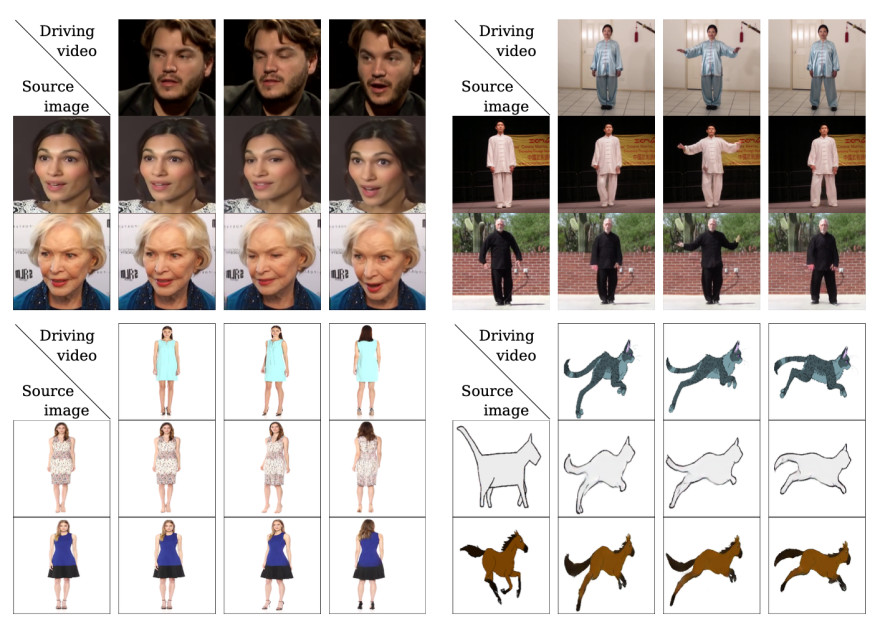Figure 1 from the paper.

Of course we want to keep the appearance of the object in the source image. For example the same face or human body.

In order to achieve this, the authors of this paper used self-learned keypoints and local affine transformations to model complex motions. A generator model takes the appearance of the source image and these motions to generate a new image.

Through this post, we will dig into multiple models used for different tasks that at the end will come together to generate our new image.

Furthermore, this paper contains a lot of formulas related to calculus and, in specific, Taylor Expansion or Taylor Series. These formulas are well described and are relatively easy to understand once we know about the Taylor series. Thus, we will review the Taylor series.

The original code of this project is available on GitHub. The authors used PyTorch to create and train the models. Here we will use TensorFlow to replicate the models and the training flow. Additionally, we will review some PyTorch functions and compare these functions with the ones that TensorFlow provides.

The code of this post is available here. I will do my best to explain all the concepts needed to understand this project so I hope everything is explanatory. Let's get started.

## Optical Flow

The first step that we have to take is to learn about optical flow. If we want to generate a new image we need the motions from the driving image. These motions come in the form of a dense motion field that gives us the flow vectors of all the pixels between two consecutive frames, in this case the source and driving images. To obtain a dense motion field we use optical flow.

Optical flow is defined as the motion between two consecutive frames. We can use multiple methods to compute the optical flow. For example, we can follow the brightness constancy constraint where we assume that pixel intensities of an object are constant between consecutive frames.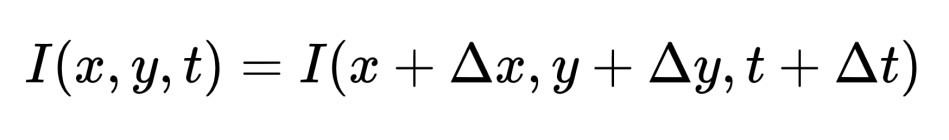We represent an image as an intensity function. We can compare I(x, y, t) to an image stored in a tensor. When we want the value of a pixel we can index the tensor like tensor_image[x, y].

Here delta x, delta y and delta t represent the movement of some pixel and time or the rate of change (delta_x = x2 - x1, ...) between the two frames. Thus, the equation represents that the intensity or color of the pixel remains the same even if it moves.

If we apply the Taylor expansion to the previous function we obtain the following equation: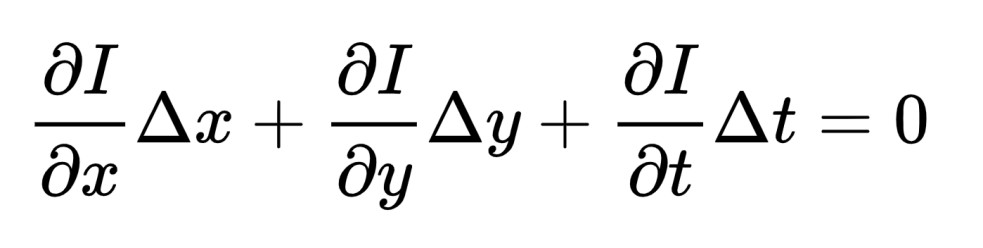Where now we have the partial derivatives of the image with respect to x, y and t `(dI/dx, dI/dy, dI/dt)` and the rate of change of x, y and t. This equation states that the change in pixel intensity is 0. Something similar to the first equation.

Our frames are separated in time by delta t. If we remember the equation of velocity:

``````v = (delta_x/delta_t) or (x2 - x1 / t2 - t1)
``````

Where velocity equals to the change in position divided by the change in time. Therefore, we can find the pixels' velocity dividing by delta t: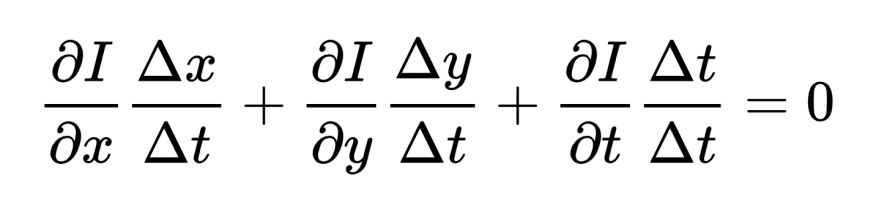Leving us with the following equation: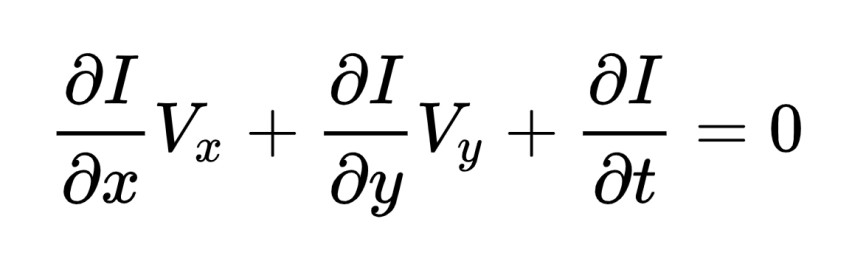Where Vx and Vy are the velocities of the pixel x, y or the optical flow that we are looking for.

We can use a different notation for the previous function: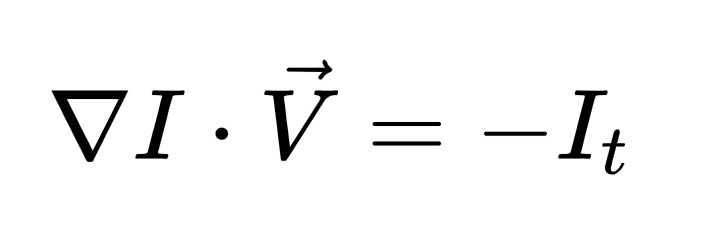.

Now I is a vector that contains the partial derivatives with respect to x and y. Thus, I is the gradient of the image. Image gradients point in the direction of the highest change in the intensity or color in the image. Since we assume that pixels' intensities or colors are constant between frames, when we move a pixel we have a change in the intensity, using the image gradient we can find the direction of that change. Also, V is now a vector that contains the velocity in both x and y directions. When we multiply these two vectors we obtain the negative rate of change of the image with respect to the time.

In other words, in order to compute the optical flow between two frames we need to calculate the velocity in both directions of the pixels. Image gradient already gives us the direction and we can compute this gradient using a convolution kernel. We can say that the function above works as a template.

One interesting property is that the velocity values Vx and Vy of some pixel lie somewhere on the direction of the gradient of that pixel. We can see this as looking for a value on a line.

We will use optical flow to find out the dense motion field between our images. We won't use the brightness constancy constrain, however, the equation that we will use follows the same principles than the equations that we have seen. Thus, to have a further understanding, we will learn about Taylor expansion.

### Taylor Series

We can use the Taylor Expansion to approximate a function using the function's derivatives at a single point. The formula to build this approximation is: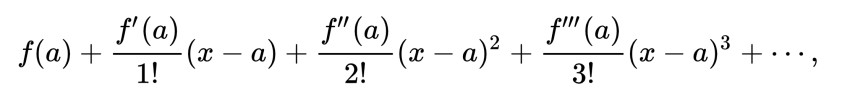Where a denotes the point around where the terms are evaluated and the functions f', f'', ... are the derivatives of the original function. Let's see how we can expand or approximate the cosine function.

In essence, we have to compute the derivatives of the cosine function depending on the approximation that we want. For instance, if we want a first order approximation we compute the first derivative of the cosine function, if we want a second order approximation, we also have to compute the second derivative and so on. As we compute more derivatives, the expansion approximates better the original function. Let's compute the first 3 derivatives of the cosine function:

``````f(x) = cos(x)

f'(x) = -sin(x)
f''(x) = -cos(x)
f'''(x) = sin(x)
``````

Now we can start building a polynomial using these derivatives like in the previous formula:

``````cos(a) = cos(a) - (sin(a) / 1!)(x - a) - (cos(a) / 2!)(x - a) ^ 2 + (sin(a) / 3!)(x - a) ^ 3
``````

To obtain the final polynomial we have to choose a value for a and evaluate the functions using this value. The value for a is choosen depending around where we want the approximation. For instance, if we choose 0 the approximation will be good predicting the values of the original function around 0. When we choose 0 the expansion is called Maclaurin series.

``````When a = 0

cos(0) = 1
sin(0) = 0

cos(0) = 1 - (0 / 1!)(x - 0) - (1 / 2!)(x - 0) ^ 2 + (0 / 3!)(x - 0) ^ 3

Simplify:

cos(x) = 1 - (x ^ 2/ 2!)

``````

In the latter equations we can notice some interesting properties of these approximations. The first term of the equation is a constant that we get evaluating the original function at a. In fact, if we only use the first term to approximate a function, this approximation is called zeroth approximation and it's just a straight line with slope 0. When we use two terms or a first order approximation, we are also adding the first derivative of the original function. This derivative is the slope of the function. Thus, now we have an equation like:

``````f(a) + (f'(a) / 1!)(x - a)

since 1! equals to 1:

f(a) + f'(a)(x - a)

Assign some value to a:

f(0) + f'(0)x
``````

You may notice that this equation is the equiation of a line:

``````b + mx
``````

Where m is the slope and b is the y-intercept. Therefore, a first order approximation is a linear approximation of the original function. The authors in the paper mention that a previous paper used a zeroth approximation of the motion between the source and driving images. However, sometimes this approximation leads to poor results, Thus, the authors of this paper made a linear approximation of the motion to improve the results.

In the following image we can see the approximations of the sine function. As we add more high order derivatives, the polynomial approximates better the original function (black line):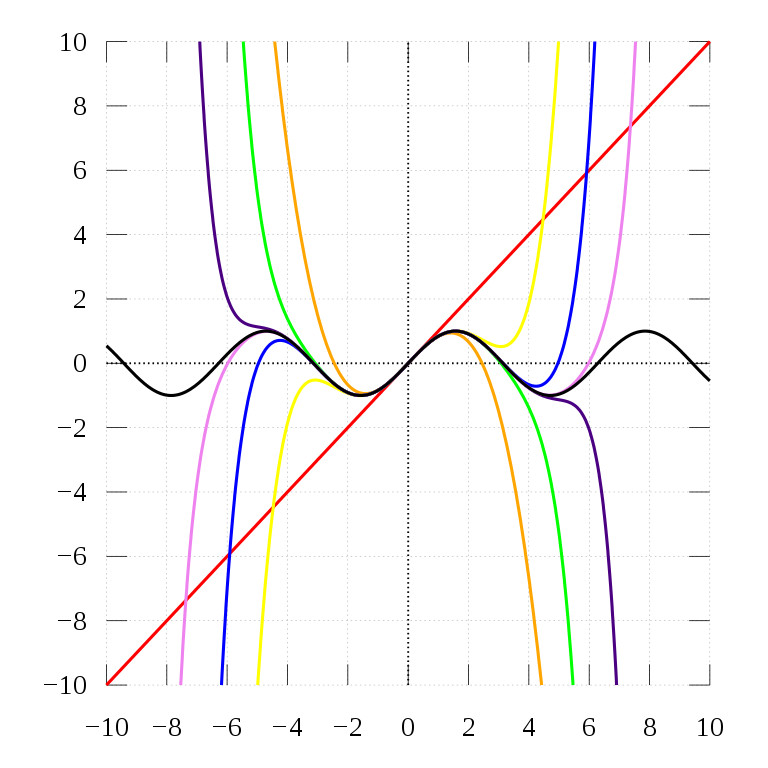Image from Wikipedia

Having seen how the Taylor expansion works, let's see how we expand the brightness constancy constraint.

As we know, we assume that pixel intensities of an object are constant between consecutive frames. In addition, we have a second assumption, the distance between the two frames is really small. This suggests that we only care about the values around a small part of the function and we don't need the complete function. Then, we can linearize the intensity function and get a simpler function to work with.

This can be done by expanding the function using a first order or linear approximation. In this way, we also add the partial derivatives or gradient of the image with respect to x and y to the equation. Since we only care about the values around x and y, a linear approximation is enough to find out x + dx and y + dx. Also using a line we constrain the velocity values to lie along this line.

Let's change a little bit the image intensity function:

``````I(x, y, t) = I(x + disX, y + disY, t + disT)
``````

Now we represent delta as dis. To linearize this function we apply the Taylor expansion to the right side of the equation:

``````I(x, y, t) = I(x, y, t) + (dI/dx)disX + (dI/dy)disY + (dI/dt)disT
``````

In this case we have three variables so we have to compute three derivatives. We evaluate the functions at x, y, t, like we did with a in the cosine example, also we simplify the subtraction (x - a) or in this case (disX - x), (disY - y), (disT - t) as disX, disY and disT.

We can cancel `I(x, y, t)`:

``````I(x, y, t) -  I(x, y, t) = (dI/dx)disX + (dI/dy)disY + (dI/dt)disT
0 = (dI/dx)disX + (dI/dy)disY + (dI/dt)disT
``````

Now we divide by disT:

``````(dI/dx)(disX/disT) + (dI/dy)(disY/disT) + (dI/dt)(disT/disT) = 0
``````

We end up with two important terms:

``````(disX/disT), (disY/disT)
``````

This is the distance of x and y divided by the time, and as we remember this is the equation of velocity. We can substitute these two terms and use the variables Vx and Vy that now represent the velocity in the x and y directions and obtain the final equation:Once we have seen what optical flow is and how we expand it using Taylor expansion, let's check how the authors represented the motion between frames.

## Keypoints and Local Affine Transformations to model Complex Motions

As mentioned earlier, we will compute a dense motion field to get the motions between the source image, which we can refer to as S and the driving image, which we can refer to as D. These motions are used by the generator to create a new image with the same motions. The dense motion field is modeled by the function:

``````TS <- D
``````

That maps each pixel location in D with its corresponding location in S. This function is referred as backward optical flow where we sample new pixels using a differiantable method called bilinear interpolation so the gradients can flow from the loss function through the network. Further in this post, we will learn about bilinear interpolation and we will use it to align the generated image with respect to the pixels of the driving image.

In order to get the dense motion field function we assume there exists an abstract reference frame R. Using R we can independently estimate two transformations: from R to S:

``````TS <- R
``````

and from R to D:

``````TD <- R
``````

The authors made this choice so we can estimate S and D independently. Thanks to this, the estimation is easier when the source and driving images are different visually.

Also, the frame R is an abstract concept that cancels out in further derivations. The authors mention that we can not visualize this frame. However, we can get a visual idea of how it's used later in this section.

`TS <- R` and `TD <- R` are keypoints in the source and driving images predicted by a keypoint detector network. We will see this network in the next section.

As stated in the paper, the keypoint representation acts as a bottleneck resulting in a compact motion representation. We can say that this is a latent representation of the motion.

The keypoint detector network also outputs the parameters of affine transformations. These transformations model motion around each keypoint. Thus, we model the motion using these keypoints and affine transformations.

Since `TS <- D` is used to model the dense motion field. We use Taylor expansion to represent `TS <- D` by a set of keypoint locations and affine transformations. We will see this more clearly in the following equations.

To fully understand this, the authors in the paper put an example using a random frame X, in the following equations we can replace X with S or D. Given a transformation:

``````TX <- R
``````

We compute its first order Taylor expansions (Approximate the function linearly) in K points p1,...pk (Thus, we have a expansion around each keypoint). Here p1,...pk denotes the coordinates of the keypoints in R: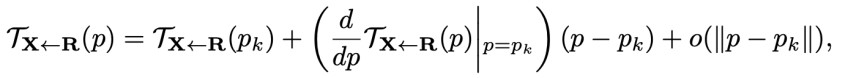Equation 1 in the paper.

The equation above is the Taylor expansion of `TX<-R` where: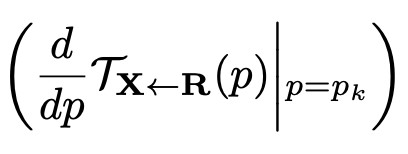Is the derivative of `TX<-R` evaluated around pk and the second term of the Taylor expansion (Here pk is used as a in the original Taylor series function).

Here we only show one Taylor expansion where P represents all the keypoints p1,...pk in the frame R.

We can ignore the last term of the equation, this term is called little o and shows around where we approximated the Taylor expansion, in this case around (p - pk).

The final equation containing all the Taylor expansions looks like: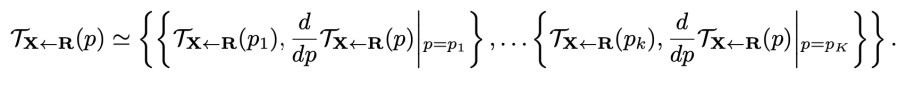Equation 2 in the paper.

Since we have a multivariable function `TX<-R` that depends of two variables x and y (pk = (x, y)), the derivative of this function is a matrix of gradients, also called Jacobian Matrix.

The Jacobian is a matrix of first order partial derivarives. In my previous post, I talked about how we can see a matrix multiplication as a transformation. `TX<-R` is a function that moves the location of the keypoints of R to the location of the keypoints in X. Seen in a different way, internally `TX<-R` is transforming the matrix of keypoints [x, y] using a matrix multiplication, in this multiplication we multiply by a new matrix that contains the transformations needed to move R to X, like translations or rotations.

The Jacobian matrix works in a similar way. The partial derivatives of this matrix describe how the neighborhood of x and y is transformed by `TX<-R`. Thus, computing the Jacobian matrix we can get the local affine transformations around a keypoint.

As we are expanding the `TS <- D` function, we are also adding its local transformations to the equation in the form of the Jacobian matrix.

Since the dense motion field `TS <- D` maps each pixel location in D with its corresponding location in S, estimating `TS <- D` consists in estimating `TS <- R` and `TD <- R`. The equation for `TS <- D` is: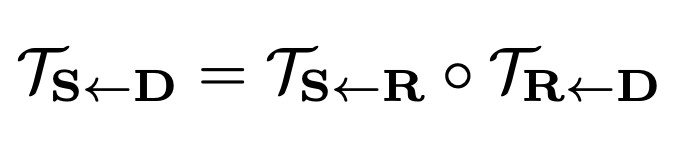Equation 3 in the paper.

This equation consists in a matrix multiplication between `TS<-R` and `TR<-D`.

We can visualize the relation between S, R and D as: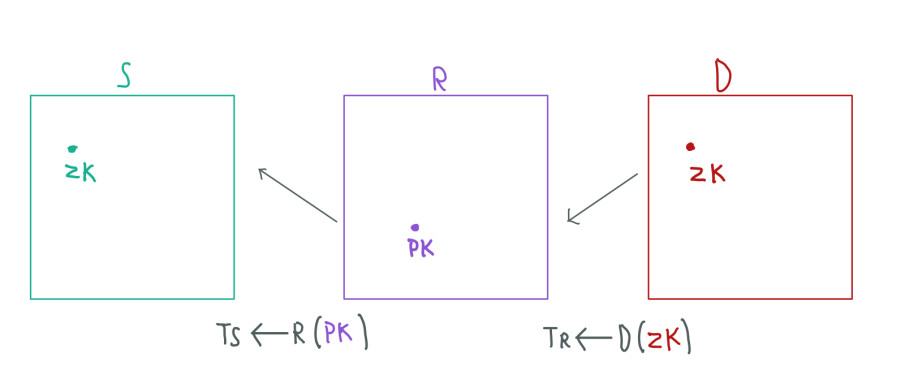Zk represents the keypoint location in S or D whereas Pk represents the keypoint location in R

Where:

``````Pk = TR<-D(Zk)
``````

Thus, we can get the keypoint location Pk in R estimating `TR<-D` at Zk of D, given that Zk is the pixel location corresponding to the keypoint location Pk in R. Also:

``````Zk = TS<-R(Pk)
``````

Where this Zk is the keypoint location in S. In this way we can get `TS<-D` estimating these two transformations.

As we can notice, we need a transformation `TR<-D`. However, this is not possible since R is just an abstract concept and is not computed. Using X as an example frame again we can say that: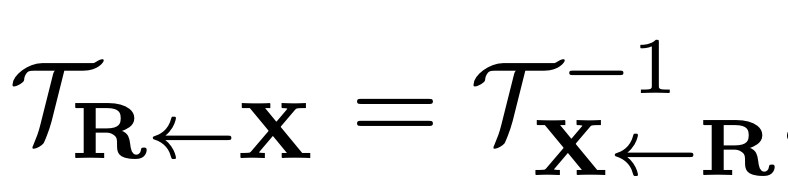The transformation from X to R is the same as the inverse of the transformation from R to X. This equality is possible when `TX<-R` is locally bijective in the neighbourhood of each keypoint. This means, that each keypoint of R is mapped to each keypoint of X.

As we saw, the keypoint detector network outputs `TD<-R`, so we only need to invert this transformation. The final equation to obtain the dense motion field looks like: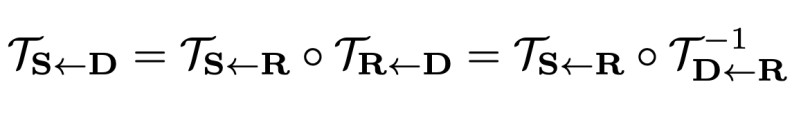Complete equation 3 in the paper.

When we apply the Taylor expansion to this equation we end up with the following equation: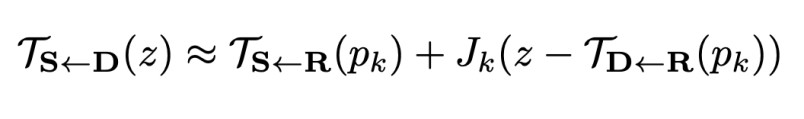Equation 4 in the paper. Z represents all the keypoint locations in S and D.

Where the Jacobians Jk are: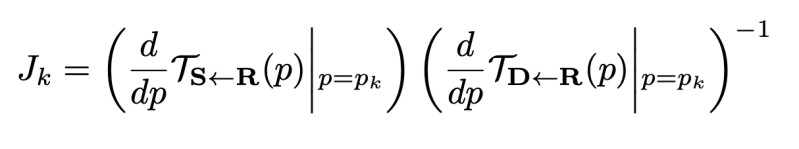We can find the math behind the following steps in the supplementary material at the end of the paper in section A.1. So, let's apply this expansion step by step:

Firstly, we have to take the following equation as a template: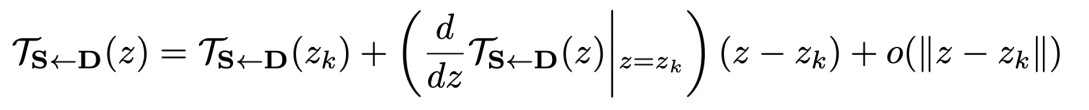In a similar way that we did with the cosine example:

``````cos(x) = cos(a) - (sin(a) / 1!)(x - a) or
cos(x) = cos(a) - sin(a)(x - a)
``````

Here we only take into account a first order expansion.

In this template the first and second term represent the original function and its first derivative evaluated at Zk respectively. In the third term we have (Z - Zk) as (x - a). We can ignore the fourth term.

To compute the zeroth order expansion, we have to evaluate `TS<-D` at Zk.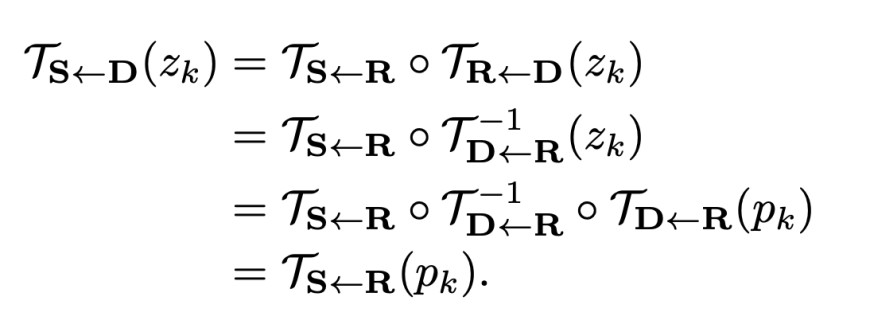The equation above tells us that:

We obtain `TS<-D` computing `TS<-R . TR<-D` or `TS<-R . T-1D<-R`. We know that:

``````TR<-D = T-1D<-R

where

Pk = TR<-D(Zk) = T-1D<-R(Zk)
``````

Estimate the transformation TR<-D(Zk) or T-1D<-R(Zk) near Zk gives us Pk.

Thus, we also have that:

``````T-1R<-D = TD<-R
``````

Then:

``````Zk = TD<-R(Pk) (Zk of D)
``````

Estimate the transformation TD<-R(Pk) near Pk gives us Zk of D.

So we can write:

``````T-1D<-R(Zk) = T-1D<-R . TD<-R(Pk)
``````

and write:

``````pk = T-1D<-R . TD<-R(Pk)
``````

Finally we end up with only one term:

``````TS<-R(pk)
``````

This computation can be confusing, so let's imagine that as we compute `TS<-D` we relize we can reduce the equation and only use one term.

To compute the first order expansion we have to differentiate `TS<-D` that contains two functions, `TS<-R` and `T-1D<-R`. Thus, we have to use the composition rule also know as the chain rule.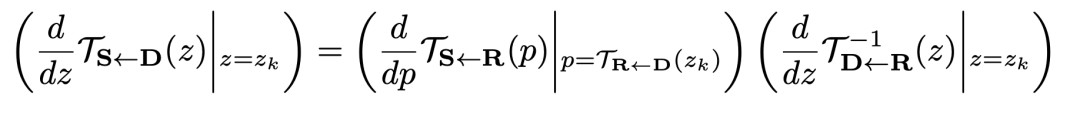Using the chain rule we compute the derivative of `TS<-R` and `T-1D<-R`. However, we can use the inverse of the Jacobian matrix since the latter is equal to the Jacobian of the inverse function:

``````J(f-1) = J-1(f)
``````

Thus, we get: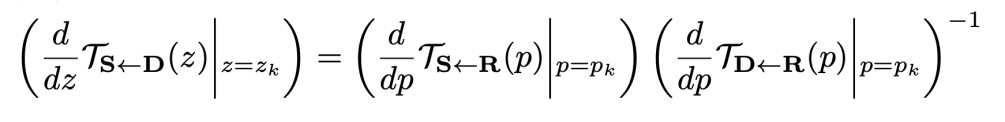Our final equation after the Taylor expansion is: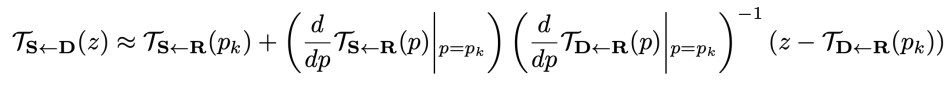Where:

``````TS<-R(pk) Keypoints of the source image
d/dp TS<-R(p) Jacobians of the source image
d/dp TD<-R(p) Jacobians of the driving image
``````

There are more math coming further in this post. By now, this is all the math that we need to know to start looking at the code.

## Networks

This work contains several networks. To understand how all these networks are connected, let's see the following scheme: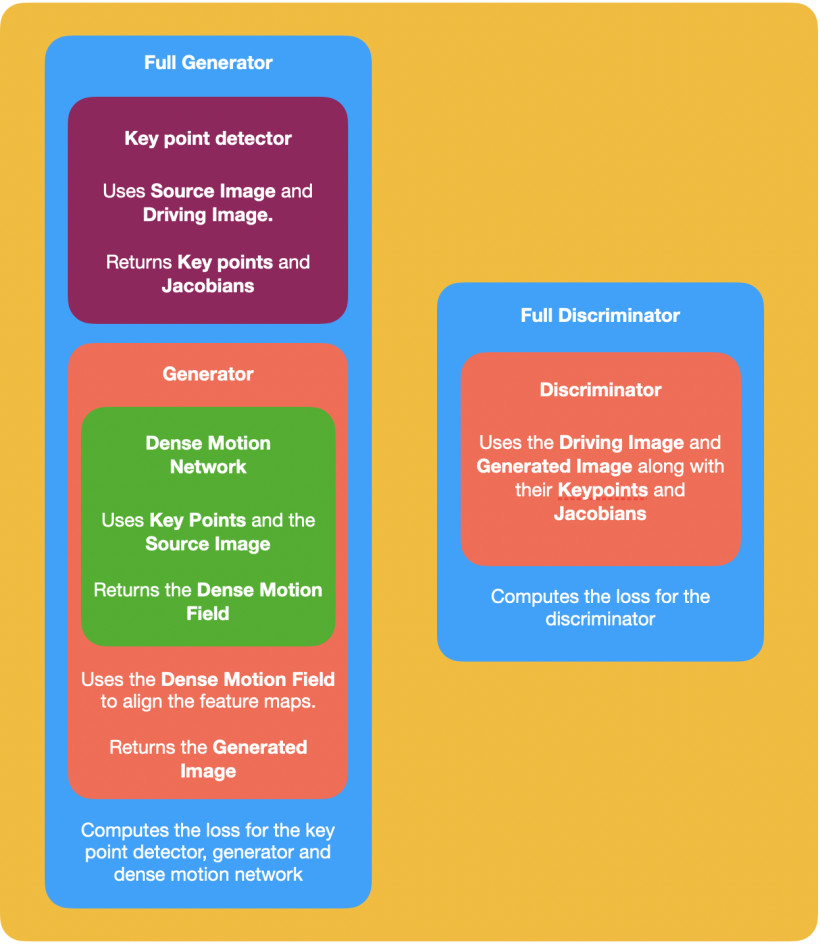We have two main models, a Full Generator and a Full Discriminator. Inside these models we call all the other networks. We use the outputs of some of these networks as inputs of the next networks.

## Keypoint Detector Network

This network follows the U-Net architecture. In the original code the authors call this model Hourglass. I guess this was done since this name has been given to several architectures that compute landmark locations. In the paper called Unsupervised Discovery of Object Landmarks as Structural Representations we can find this name. In the latter, the Hourglass model outputs confidence maps to compute the locations of the keypoints. Here we will do something similar using gaussian heatmaps:

``````class KeypointDetector(tf.keras.Model):
def __init__(self):
super(KeypointDetector, self).__init__()
self.scale_factor = 0.25
self.num_jacobian_maps = 10
self.num_keypoints = 10
self.num_channels = 3
self.down_features_list = [64, 128, 256, 512, 1024]
self.up_features_list = [512, 256, 128, 64, 32]
self.num_blocks = 5
self.temperature = 0.1

self.predictor = Hourglass(self.down_features_list, self.up_features_list, self.num_blocks)
self.keypoints_map = layers.Conv2D(self.num_keypoints, (7, 7), strides=1, padding='valid')

# Initialize the weights/bias with identity transformation localisation network
weigth_initializer = tf.keras.initializers.zeros()
bias_initializer = tf.keras.initializers.constant([1, 0, 0, 1] * 10)
self.jacobian = layers.Conv2D(self.num_keypoints * 4, (7, 7), strides=1, padding='valid', bias_initializer=bias_initializer, kernel_initializer=weigth_initializer)

self.down = AntiAliasInterpolation(self.num_channels, self.scale_factor)

def get_gaussian_keypoints(self, heatmap):
heatmap = tf.expand_dims(heatmap, -1)
grid = make_coordinate_grid(heatmap.shape[1:3], heatmap.dtype)
grid = tf.expand_dims(grid, axis=2)
grid = tf.expand_dims(grid, axis=0)

value = heatmap * grid
value = tf.keras.backend.sum(value, axis=[1, 2])

kp = {'value': value}

return kp

def call(self, x):
model = self.down(x)
feature_map = self.predictor(model)
raw_keypoints = self.keypoints_map(feature_map)

final_shape = raw_keypoints.shape

heatmap = tf.keras.activations.softmax(raw_keypoints / self.temperature, axis=[1, 2])
final_keypoints = self.get_gaussian_keypoints(heatmap)

jacobian_map = self.jacobian(feature_map)

jacobian_map = tf.reshape(jacobian_map, [final_shape, final_shape, final_shape, self.num_jacobian_maps, 4])

heatmap = tf.expan_dims(heatmap, axis=-1)

jacobian = heatmap * jacobian_map

jacobian = tf.reshape(jacobian, [final_shape, -1, final_shape, 4])

jacobian = tf.keras.backend.sum(jacobian, axis=1)

jacobian = tf.reshape(jacobian, [jacobian.shape, jacobian.shape, 2, 2])

final_keypoints['jacobian'] = jacobian

return final_keypoints
``````

I suggest you to check the code as you follow the post since the code has comments and more implementations for some models that we don't see here due to the fact that they are not that important. For example, the Hourglass code implementation that is just a U-Net model.

If you are also checking the original code, remember that PyTorch uses by default the order batch x channels x height x width for its tensors and TensorFlow uses the order batch x height x width x channels. Thus, some computations need a re-order of dimensions.

### Keypoints

To get the keypoints from an image, the KeypointDetector does the following:

We add a convolutional layer with 10 filters to the output of the Hourglass model. Each filter represents a keypoint. Therefore, the output of this convolutional layer are feature maps of size batch x height x width x 10.

To get the locations of the keypoints in the feature maps we get the location of the maximum values of each feature map. Normally, we would use the argmax function to get the position of the maximum value in an array or matrix. However, this funcion is not differentiable (we can not compute its gradients). Hence, we will use a similar function called soft-argmax that is differentiable.

First, we increase the values in the feature maps by dividing by some decimal value (0.1). With this, the soft-argmax function is easer to compute. We apply the softmax function along the spatial axes (height, width) so we get a confidence map where the sum of the pixels of each feature map is 1.

The method get_gaussian_keypoints does the rest of the job. The soft-argmax method is showed in the paper called Laplace Landmark Localization.pdf where it's used to localize landmarks as well. You can see a description and the formula (2) in section 3.1 of the paper. Inside the get_gaussian_keypoints method we call an important and simple function called make_coordinate_grid. This function creates a grid of the specified size which values goes from -1 to 1. This function will be used a lot further in the post.

Finally, the final_keypoints variable has a shape of batch x 10 x 2 where each keypoint has two values, the x and y positions.

### Jacobians or Affine Transformations.

To get the Jacobians from an image, the KeypointDetector does the following:

We add a convolutional layer with 40 filters to the output of the Hourglass model. This convolution is intialized with weights and bias that follow the identity transformation (A transformation that returns the original matrix).

The idea behind this initialization comes from the Spatial Transformer Networks where we have a module called Localisation net that outputs the affine transformations of the object in an image. Thus, initializing this layer using an identity transformation leads to obtain the affine transformation of the object. We can use the output of this layer to get the affine transformations instead of computing the Jacobian matrix, which would be computationally expensive.

We need 40 filters since each Jacobian needs 4 parameters. To get the final parameters for the affine transformations we compute the spatial weighted average of these filters using as weights the keypoints' confidence map. The final jacobian variable has a shape of batch x 10 x 2 x 2.

We also have an AntiAliasInterpolation function that, as its name suggests, applies an anti-alias kernel using one convolutional layer and then reduces the size of the input image. The values for the kernels are not learned but specified when computed.

In TensorFlow, we have a native function to reduce the size of an image and apply an anti-alias kernel. However, this function returns a different result, so I opted to re-create the AntiAliasInterpolation function like in the original code.

Further in the post, we will see how we can train this network. We need a special loss function since the network learns to recognize keypoints from an image in a self-supervised way. Thus, we don't need keypoint locations or any kind of label.

## Dense Motion Network

Here, I will only show the most important parts of the network. I recommend you to follow the complete code to fully understand what the network does.

``````self.hourglass = Hourglass(self.down_features_list, self.up_features_list, self.num_blocks)
self.mask = layers.Conv2D(self.num_keypoints + 1, (7, 7), strides=1, padding="valid")
self.occlusion = layers.Conv2D(1, (7, 7), strides=1, padding="valid")
self.down = AntiAliasInterpolation(self.num_channels, self.scale_factor)
``````

This network uses the Hourglass model as principal architecture. The mask and occlusion layers will be used on the top of the hourglass model.

``````def call(self, source_image, kp_driving, kp_source):
source_image = self.down(source_image)
image_size = source_image.shape
batch_size, height, width, _ = image_size
out_dict = dict()

heatmap_representation = self.create_heatmap_representations(image_size, kp_driving, kp_source)
sparse_motion = self.create_sparse_motions(image_size, kp_driving, kp_source)
warped_images = self.create_deformed_source_image(source_image, sparse_motion)

input = tf.concat([heatmap_representation, warped_images] axis=-1)
input = tf.permute(input, [0, 2, 3, 1, 4])
input = tf.reshape(input, [batch_size, height, width, -1])

prediction = self.hourglass(input)
``````

In the original code we return a lot of examples for debug purposes. Here I avoid them to make the code shorter.

To call this model we need the source image, its keypoints (kp_source) as well as the keypoints of the driving image (kp_driving). The main model self.hourglass takes as input concatenated heatmaps and transformed images.

We use the heatmaps to indicate the model where the keypoints and transformations happens. This information is also useful to predict occlusion maps. We implement these heatmaps as the difference of two heatmaps centered in `TD<-R(Pk)` and `TS<-R(Pk)` (The keypoints from D and S).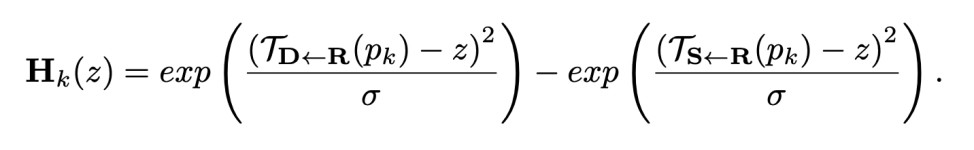Equation 6 in the paper.

This implementation is done in the create_heatmap_representations function:

``````def create_heatmap_representations(self, image_size, kp_driving, kp_source):
spatial_size = image_size[1:4]

gaussian_driving = keypoints_to_gaussian(kp_driving, spatial_size=spatial_size, kp_variance=self.kp_variance)
gaussian_source = keypoints_to_gaussian(kp_source, spatial_size=spatial_size, kp_variance=self.kp_variance)

heatmap = gaussian_driving - gaussian_source

zeros = tf.zeros((heatmap.shape, 1, spatial_size, spatial_size), dtype=heatmap.dtype)

heatmap = tf.concat([zeros, heatmap], dim=1)
heatmap = tf.expand_dims(heatmap, axis=-1)

return heatmap
``````

Where we call the function keypoints_to_gaussian:

``````def keypoints_to_gaussian(keypoints, spatial_size, kp_variance):
# TD<-R or TS<-R in equation (6)
mean = keypoints["value"]

# Z in equation (6)
coordinate_grid = make_coordinate_grid(spatial_size, mean.dtype)
coordinate_grid = tf.expand_dims(tf.expand_dims(coordinate_grid, axis=0), axis=0)

repeats = mean.shape[:2] + (1, 1, 1)
coordinate_grid = tf.tile(coordinate_grid, multiples=repeats)

shape = mean.shape[:2] + (1, 1, 2)

mean = tf.reshape(mean, shape)

mean_sub = (mean - coordinate_grid)

out = tf.exp(-0.5 * tf.keras.backend.sum(mean_sub ** 2, axis=-1) / kp_variance)

return out
``````

We can notice that this function just computes the equation 6. We can visualize the output of this function as: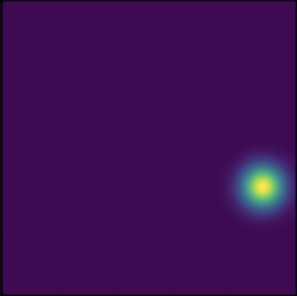This is just a guassian heatmap around the keypoint location. We subtract the heatmaps from `TD<-R(Pk)` and `TS<-R(Pk)` to get H(z).

We also add a heatmap full of zeros to represent the background keypoints (no keypoints):

``````zeros = tf.zeros((heatmap.shape, 1, spatial_size, spatial_size), dtype=heatmap.dtype)
``````

The Dense Motion Network estimates the dense motion field from the set of Taylor approximations of `TS<-D` and the original source image S. The problem here is that the local patterns in `TS<-D`, such as edges or textures, are pixel-to-pixel aligned with D, since the transformations come from D to S. This misalignment makes the task harder for the network to predict the dense motion field from S. To overcome this, we can align the source image warping S according to the transformations estimated in equation 4:

``````sparse_motion = self.create_sparse_motions(image_size, kp_driving, kp_source)
warped_images = self.create_deformed_source_image(source_image, sparse_motion)
``````

First we need to compute the motions:

``````def create_sparse_motions(self, image_size, kp_driving, kp_source):
batch_size, height, width, _ = image_size
# Z in equation (4)
identity_grid = make_coordinate_grid((height, width), type=kp_source['value'].dtype)
identity_grid = tf.expand_dims(tf.expand_dims(identity_grid, axis=0), axis=0)
# shape 1 x 1 x 256 x 256 x 2

# TD<-R in equation (4)
driving_keypoints = kp_driving['value']
shape = driving_keypoints.shape[:2] + (1, 1, 2)
driving_keypoints = tf.reshape(driving_keypoints, shape)
# shape batch x 10 x 1 x 1 x 2

# Z - TD<-R in equation (4)
coordinate_grid = identity_grid - driving_keypoints
# shape batch x 10 x 256 x 256 x 2

# Using the inverse of d/dp Td <- R ; Equation (5) Jk
jacobian = tf.linalg.matmul(kp_source['jacobian'], tf.linalg.inv(kp_driving['jacobian']))
# shape batch x 10 x 2 x 2

jacobian = tf.expand_dims(tf.expand_dims(jacobian, axis=-3), axis=-3)
jacobian = tf.tile(jacobian, [1, 1, height, width, 1, 1])
# shape batch x 10 x 256 x 256 x 2 x 2

# Jk . (Z - TD<-R) in equation (4)
coordinate_grid = tf.linalg.matmul(jacobian, tf.expand_dims(coordinate_grid, axis=-1))
coordinate_grid = tf.squeeze(coordinate_grid) # remove last axis
# shape batch x 10 x 256 x 256 x 2

source_keypoints = kp_source['value']
# shape batch x 10 x 2

shape = source_keypoints.shape[:2] + (1, 1, 2)
source_keypoints = tf.reshape(source_keypoints, shape)
# shape batch x 10 x 1 x 1 x 2

# Ts <- D(z) where source_keypoints is TS<-R and coordinate_grid is Jk . (Z - TD<-R)
driving_to_source = source_keypoints + coordinate_grid
# shape batch x 10 x 256 x 256 x 2

# Adding background feature, background feature is just the identity_grid without motions
identity_grid = tf.tile(identity_grid, [batch_size, 1, 1, 1, 1])
# shape batch x 1 x 256 x 256 x 2

sparse_motions = tf.concat([identity_grid, driving_to_source], dim=1)
# shape batch x 11 x 256 x 256 x 2

return sparse_motions
``````

You may notice that the operations in the function above follow the terms in equation 4. In other words, we are building the Taylor expansion using the Keypoints and Jacobians from the source (kp_source) and driving (kp_driving) images. You can also notice that we used the make_coordinate_grid function and we reshape tensors by adding or removing dimensions so the tensors' shape match and the operations can be computed. Basically, we are adding the motions of `TS<-D` to the identity_grid to build the sparse_motions tensor.

The sparse_motions tensor has a shape of batch x 11 x 256 x 256 x 2. As we know, we have one Taylor expansion for each keypoint. We have 10 keypoints and one background representation. We can notice that we have a tensor where we repeated the source image 11 times. We will warp this tensor according with each motion in the sparse_motions tensor. Thus, we will move all the pixels of each image according with each motion

Each location in the sparse_motions tensor that we can see as a grid indicates where the pixels values should come from. For instance:

``````sparse_motions[25, 26] = [27, 28]
``````

Here we are saying: for the first image in the batch and for the second motion, the pixel values in the location [25, 26] should come from the pixel values in the location [27, 28]. This can be seen as, move the pixels from [27, 28] to [25, 26]. Basically, we are adding the motions from the Taylor expansion to the grid that the make_coordinate_grid function returns. We should also remember that make_coordinate_grid returns a grid in a range between [-1, 1]. Then the pixel locations of these motions are also in that range:

``````sparse_motions[1, 1] = [-0.98, -0.108]
``````

Now that we have the motions, we can align the source image according with these motions:

``````def create_deformed_source_image(self, source_image, sparse_motions):
batch_size, _, height, width = source_image.shape
# batch x 256 x 256 x 3

source_repeat = tf.expand_dims(tf.expand_dims(source_image, axis=1))
source_repeat = tf.tile(source_repeat, [1, self.num_keypoints + 1, 1, 1, 1])
source_repeat = tf.reshape(source_repeat, [batch_size * (self.num_keypoints + 1), height, width, -1])
# (batch . 11) x 256 x 256 x 3

sparse_motions = tf.reshape(sparse_motions, [batch_size * (self.num_keypoints + 1), height, width, -1])
# (batch . 11) x 256 x 256 x 2

new_max = width - 1
new_min = 0
sparse_motions = (new_max - new_min) / (tf.keras.backend.max(sparse_motions) - tf.keras.backend.min(sparse_motions)) * (sparse_motions - tf.keras.backend.max(sparse_motions)) + new_max

sparse_deformed = tfa.image.resampler(source_repeat, sparse_motions)
# (batch . 11) x 256 x 256 x 3

sparse_deformed = tf.reshape(sparse_deformed, [batch_size, (self.num_keypoints + 1), height, width, -1])
# batch x 11 x 256 x 256 x 3

return sparse_deformed
``````

In this function we apply a bilinear interpolation using the function tfa.image.resampler to the source_repeat variable that contains our source image repeated 11 times so we can apply the 11 motions using the sparse_motions tensor.

The tfa.image.resampler function comes from the TensorFlow addons package, this function recieves an input of shape batch x height x width x channels and a grid of shape batch x height x width x 2. That's why we have to combine the first and second axis batch . 11 so we can use our tensors in this function.

In PyTorch we have a more powerfull function already added in the framework called torch.nn.functional.grid_sample this function also needs inputs of the same shape and the grid input has to be in range [-1, 1]. Due to this, our make_coordinate_grid returns a grid in that range. In contrast the input grid of the tfa.image.resampler function needs to be in a range [0, width - 1]. Although this is not specified in the documentation, I tried to use this function with a grid in the range [-1, 1] but it didn't work so we have to change the range of the grid:

``````new_max = width - 1
new_min = 0
sparse_motions = (new_max - new_min) / (tf.keras.backend.max(sparse_motions) - tf.keras.backend.min(sparse_motions)) * (sparse_motions - tf.keras.backend.max(sparse_motions)) + new_max
``````

### Bilinear Interpolation

The main idea behind interpolation is to estimate new data points within a range. It's often used to transform images. For example, reduce or increase its size. And it's also used in backward warping to estimate the values of the pixels of a new image.

Let's see an example of linear interpolation: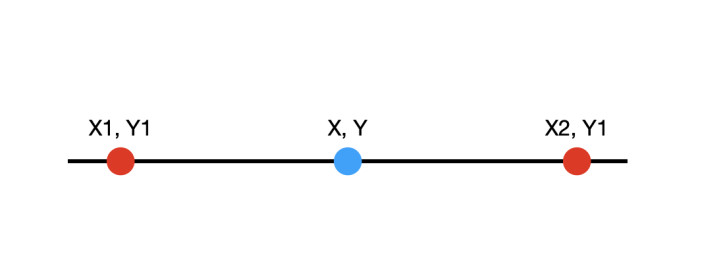We know the values of the red points and we want the value of the blue point. We can get this value using linear interpolation. Let's supose these two points are pixels in the locations:

``````x1, y1 = 35, 10
x2, y1 = 45, 10
``````

We use the same y1 value since we are only interpolating in the x axis.

Which values are:

``````image[x1, y1] = 155
image[x2, y1] = 55
``````

And we want the value of the blue point which location is:

``````x, y = 38, 10
``````

To compute the linear interpolation we have the following formula:

``````weight = (x - x1)/(x2 - x1)
interpolation = A_1 + weight * (A_2 - A_1)
``````

Where A_1 and A_2 are the values of the pixels in the first and second positions respectively. We can notice how we use a weight term, this weight gives more importance to the pixel that is closer to the pixel which value we want. In other words, we want a new pixel (x, y), this pixel will look similar to the pixels (x1, y1) and (x2, y2) but it will look more similar to the closer pixel (x1, y1).

If we use our values in the formula we have:

``````weight = (38 - 35)/(45 - 35)
interpolation = 155 + weight * (55 - 155)
``````

The variable interpolation now contains the value for the pixel (x, y).

Bilinear interpolation works in a similar way. However, we use four points instead of two to get the value for a new pixel (x, y):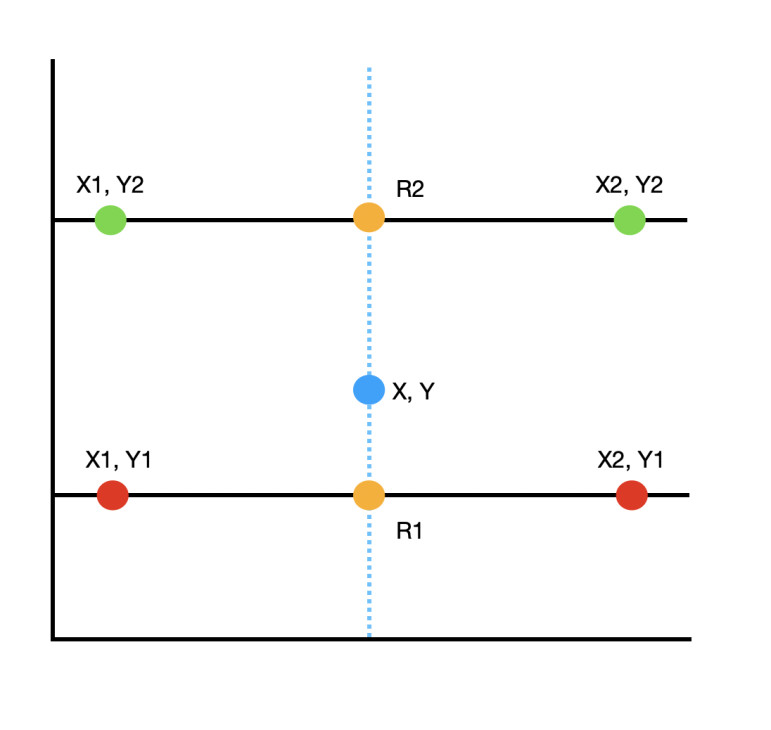The idea here is to compute three linear interpolations:

• We compute the linear interpolation between the red pixels to get the value of the yellow pixel R1.

• We compute the linear interpolation between the green pixels to get the value of the yellow pixel R2.

• Finally, we compute the linear interpolation between the yellow pixels to get the value of the blue pixel.

The first two linear interpolations are computed along the x axis and the third is computed along the y axis. Thus we have the following formula:

``````weight_1 = (x - x1)/(x2 - x1)

R1 = A_1 + weight_1 * (A_2 - A_1)
R2 = B_1 + weight_1 * (B_2 - B_1)

weight_2 = (y2 - y)/(y2 - y1)

P = R2 + weight_2 * (R1 - R2)
``````

Where A_1 and A_2 are the values for the red pixels and B_1 and B_2 are the values for the green pixels.

I would say that the hardest part about bilinear interpolation is the choose of the locations for (xn, yn). Thus, let's see an example:

Imagine that we want to resize the following image: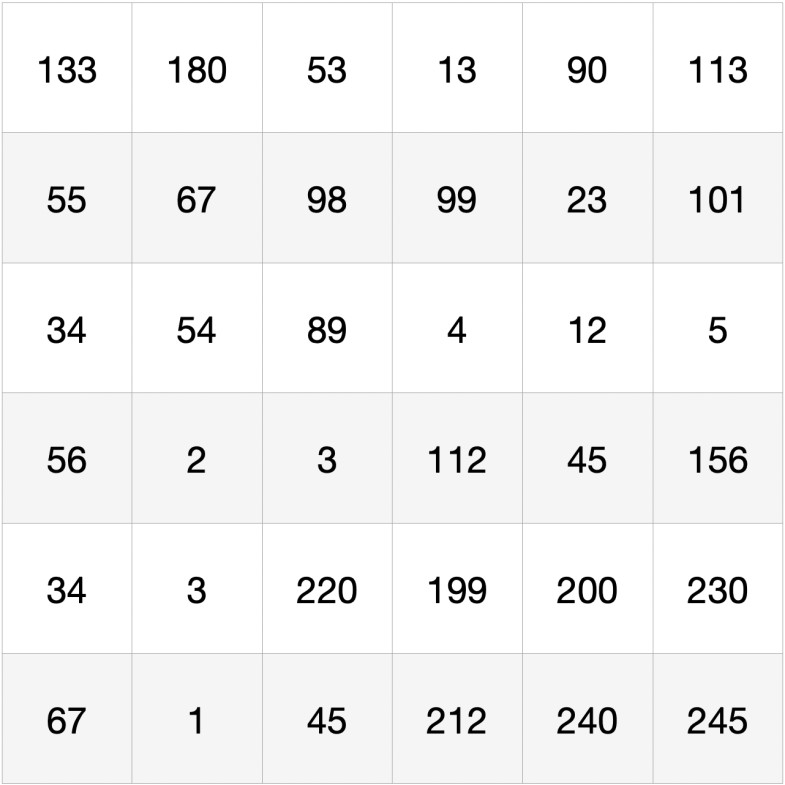From size 6x6 to 8x8. First we need to chose a range between where the pixels are, for example [-1, 1]. We can use a function like linspace to create a grid of 8 values between our range:

``````np.linspace(-1, 1, num=8)
``````

This returns the next array:

``````[-1, -0.71428571, -0.42857143, -0.14285714, 0.14285714, 0.42857143, 0.71428571, 1]
``````

These are the locations of the new pixels in both axes (x, y) that we need to interpolate to resize the image to 8x8.

We also need the six locations for the current pixels in the range [-1, 1]. We can get these locations using the same linspace function:

``````np.linspace(-1, 1, num=6)
``````

Which now returns:

``````[-1, -0.6, -0.2,  0.2,  0.6, 1]
``````

Using the first row of the image as example: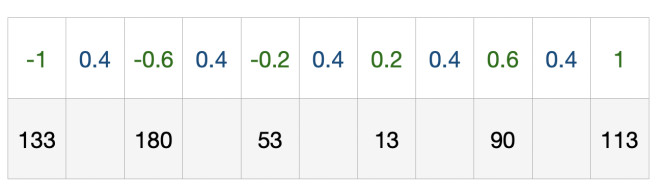We have a distance of 0.4 between pixels and the location of these pixels in the range [-1, 1]. One way of using interpolation to increase the size of an image is keeping the pixels of the edges, in this case [133, 113] and [67, 245] and interpolate all the rest pixels: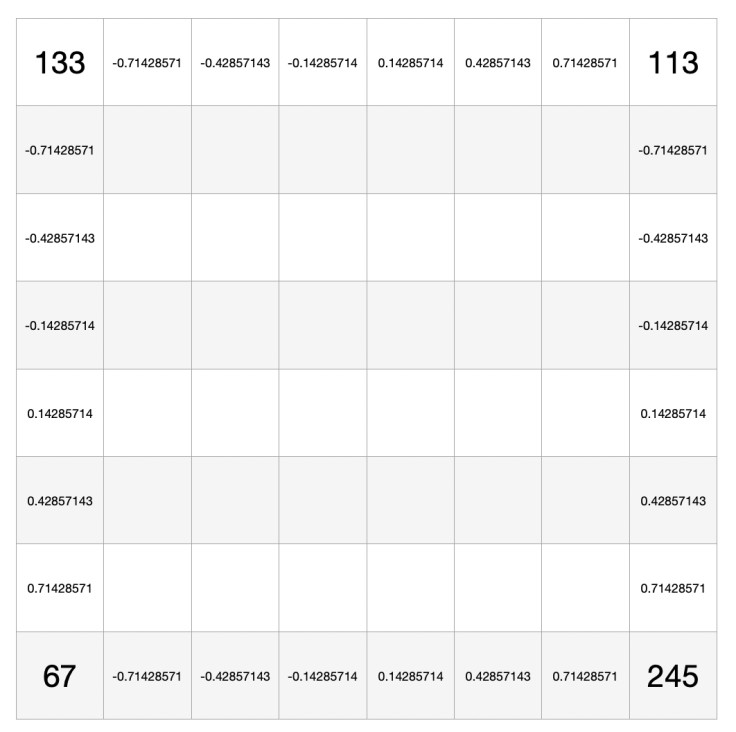For instance, if we want the value for the pixel of the third column (x = -0.42857143) and the second row (y = -0.71428571):

The x location is between -0.6 and -0.2, and the y location is between -1 and -0.6. Thus:

``````x1 = -0.6
x2 = -0.2
x = -0.42857143

weight_1 = (x - x1)/(x2 - x1)

A_1 = 67
A_2 = 98

R1 = A_1 + weight_1 * (A_2 - A_1)

B_1 = 180
B_2 = 53

R2 = B_1 + weight_1 * (B_2 - B_1)

y1 = -0.6
y2 = -1
y = -0.71428571

weight_2 = (y2 - y)/(y2 - y1)

P = R2 + weight_2 * (R1 - R2)
``````

If we want to reduce the size of some image we use a smaller linspace for the pixels that we want to interpolate.

Going back to the code, the functions torch.nn.functional.grid_sample in PyTorch and tfa.image.resampler in TensorFlow apply bilinear transformation using a grid like the one we used to represent the locations for the new pixels.

We have seen bilinear interpolation since we want to compute image warping. Image warping is used to transform or move pixels of a source image to create a new one. Image warping can be done using two methods, Forward Warping or Backward Warping.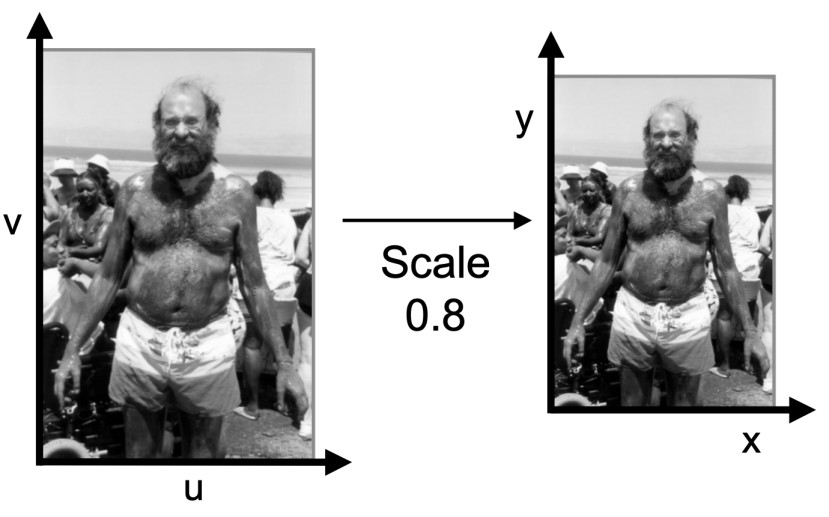You may realize that the motions `TS<-D` go from D to S but we want to move the keypoints from S to D. To explain this, we need to explore the warping methods.

In Forward Warping we iterate over the source image and we use the same pixel values but we locate them differently. In other words, each pixel in the source image is mapped to a new location in the new image:

``````Iterate over the locations u, v in the source image
for (u in source_image)
for (v in source_image)

Compute the locations for the new image
x = Fx(u, v)
y = Fy(u, v)

Copy the pixel values in u,v of the source image to the new locations in the new image
new_image[x, y] = source_image[u, v]
``````

Where the functions Fx and Fy are used to get the locations of the new pixels. For instance these functions could translate, rotate or transform u and v. Thus, let's call these functions, transformation functions.

If we could use this method to solve our problem, we would iterate over the source image S and compute the locations (x, y) using the motions from S to D `TD<-S` as the transformation functions. Therefore, the motions `TD<-S` would take as input the pixel locations of S (u, v) to get the locations of D (x, y).

Despite this method not being differentiable, it also has some problems, many pixels in the source image can map to the same destination pixel (we could repeat the same value for x and y) and some others pixels may not be covered (we could not compute some value for x and y).

In the Backward Warping (or backward optical flow) method, as its name suggests, we have the opposite behavior. We iterate over the new image, thus we cover all its pixels, and we compute the values of these pixels using bilinear interpolation:

``````Iterate over the locations x, y in the new image
for (x in new_image)
for (y in new_image)

Get the locations u, v in the source image
u = Fx-1(x, y)
v = Fy-1(x, y)

Compute the pixel values of the new image using interpolation
new_image[x, y] = interpolation(u, v)
``````

In this method we need the inverse of the transformation functions since now we need the locations of the pixels in the source image. Thus, we have that:

``````Fx(u, v) transforms u to a new location x.
Fx-1(x, y) undoes the transformation above to get the original location u.
``````

This is the reason why we compute the motions from D to S `TS<-D`. If we iterate over the driving image D, we know the locations of the pixels for the new image but we don't know how these pixels should look like. Using the motions `TS<-D` we get the locations of the pixels in S and using these locations we can compute the bilinear interpolation and get the values for the pixels.

Going back to the grid example, we had the motions `TS<-D` in the grid sparse_motions

``````sparse_motions[25, 26] = [27, 28]
``````

We used this grid in the tfa.image.resampler function to specify the locations where the function obtains the pixels values from ([27, 28]) for the pixels in the new image ([25, 26]). (The source image is in range [-1, 1] and the new image can be in range [0, length])

Bilinear interpolation is useful since the inverse transformation functions sometimes returns decimal values and not the exact pixel locations in the source image. We can also use other methods to compute the values for the pixels but here we will use interpolation.

We have seen that we had a misalignment problem since the motions `TS<-D` come from D and go to S and the source image starts in S. Warping the source image according to these motions aligns the image so it starts in D. Seen in a different way, the motions could indicate the location of an eye in D but that eye in the source image is in S. Warping the source image, we erase the eye in the S location and we repaint it in the D location and since we are using Jacobians or affine transformations we also apply transformations like rotations if any.

In order to generate the final dense motion field, we assume that the object that we want to animate (for example a human body) is composed of K rigid parts in the location of each keypoint, and each of these parts moves according with the equation `TS<-D`. These parts should behave as rigid objects that can be transformed but no deformed. Thus, the dense motion network can output masks that segment the object in K rigid parts. This trick is explored in some papers like in Optical Flow in Mostly Rigid Scenes.

``````prediction = self.hourglass(input)
# batch x height x width x 35

# batch x height x width x 11 x 1

sparse_motion = tf.transpose(sparse_motion, [0, 2, 3, 1, 4])
# batch x 256 x 256 x 11 x 2

deformation = (sparse_motion * mask)
deformation = tf.keras.backend.sum(sparse_motion, axis=3)
# batch x 256 x 256 x 2

out_dict['dense_optical_flow'] = deformation # deformation

occlusion_map = tf.keras.activations.sigmoid(self.occlusion(prediction))
# shape batch x 256 x 256 x 1

out_dict['occlusion_map'] = occlusion_map

return out_dict
``````

The self.mask layer outputs the segmentation masks for each object, including the background segmentation. We compute the element-wise product between these masks and the motions in the sparse_motion tensor. Thus, we only keep the motion in the pixels locations of each object. In the case of the background motions, we keep the original grid [-1, 1] that indicates no motion. Finally, we sum all these masked motions to form the final dense motion field of shape batch x 256 x 256 x 2. This dense motion field indicates how we have to interpolate the whole object (a human body) to have the motions of the driving image leaving the background intact.

The self.occlusion layer outputs an occlusion mask that mask out the feature maps regions that are occluded in S. We will use this mask in the generator. Thus, the generator has to infer these parts from the context.

## Generator

The architecture used to build the generator is called Johnson architecture where we use downsampling, upsampling blocks and also residual blocks.

``````def call(self, source_image, kp_driving, kp_source):

# Encoder part
for down_block in self.encoder_blocks:
out = down_block(out)

output_dict = {}

dense_motion = self.dense_motion_network(source_image, kp_driving, kp_source)

occlusion_map = dense_motion['occlusion_map']
# shape batch x 256 x 256 x 1

dense_optical_flow = dense_motion['dense_optical_flow'] # deformation
# batch x 256 x 256 x 2
out = self.deform_input(out, dense_optical_flow)
# batch x 256 x 256 x 2

if out.shape != occlusion_map.shape or out.shape != occlusion_map.shape:
occlusion_map = interpolate_tensor(occlusion_map, out)

out = out * occlusion_map

# Decoder part
out = self.bottleneck(out)

for up_block in self.decoder_blocks:
out = up_block(out)

out = tf.keras.activations.sigmoid(out)

output_dict["prediction"] = out

return output_dict
``````

The call function of the generator takes as input the source image and the keypoints and Jacobians from the source and driving images. This network follows the next steps to generate a new image:

• Encode the object appearance of the source image (encoder blocks).
• Compute the dense motion field and the occlusion mask.
• Align the feature maps of the encoded source image using the dense motion field.
• Apply the occlusion mask.
• Decode the aligned and occluded feature maps to generate the final image (decoder blocks).

To align the feature maps we use the following method:

``````def deform_input(self, x, deformation):
_, height_old, width_old, _ = deformation.shape
_ height, width, _ = x.shape

if height_old != height or width_old != width:
deformation = interpolate_tensor(deformation, width)

new_max = width - 1
new_min = 0
deformation = (new_max - new_min) / (tf.keras.backend.max(deformation) - tf.keras.backend.min(deformation)) * (deformation - tf.keras.backend.max(deformation)) + new_max

return tfa.image.resampler(x, deformation)
``````

In the deformation tensor we have the information about how we have to interpolate or align the feature maps x. Here, we use a function called interpolate_tensor to reduce or increase the size of the deformation tensor to match the size of the source image. As we have seen, we can use the function tfa.image.resampler to interpolate an image using a grid, the grid can have the same size or a smaller size than the image but it can't be bigger. Thus, inside the interpolate_tensor we pad the image to match the size of the grid and in the grid we indicate that we want to interpolate the image starting after the padding since the padding values are just zeros. Finally we use the tfa.image.resampler function to align the feature maps.

## Full Generator.

The original code of this work is made thinking about how to use multiple GPU's to train the models. Thus, the authors combine a lot of functions and loss computations inside a model called Full Generator:

``````def call(self, source_images, driving_images):
kp_source = self.key_point_detector(source_images)
kp_driving = self.key_point_detector(driving_images)

generated = self.generator(source_images, kp_source=kp_source, kp_driving=kp_driving)

loss_values = {}

pyramide_real = self.pyramid(driving_images)
pyramide_generated = self.pyramid(generated['prediction'])

perceptual_loss = 0
for scale in self.scales:
x_vgg = self.vgg(pyramide_generated['prediction_' + str(scale)])
y_vgg = self.vgg(pyramide_real['prediction_' + str(scale)])

for i, weight in enumerate(self.perceptual_weights):
loss = tf.reduce_mean(tf.abs(x_vgg[i] - tf.stop_gradient(y_vgg[i])))
perceptual_loss += self.perceptual_weights[i] * loss
loss_values['perceptual'] = perceptual_loss
``````

This model takes as input the source and driving images. The first step is to compute the keypoints and Jacobians of each image using the key_point_detector network. Then we call the generator network that we saw in the previous section using the keypoints, Jacobians and the source image to generate the image with the transfered motions.

### Perceptual Loss

Here we have a new function called ImagePyramide (self.pyramid). This function just outputs the input image at different scales or resolutions. We use this function to generate driving and generated images at different scales. Then, We use these new images in a VGG16 pre-trained network to compute the perceptual loss. This loss is used to train style transfer networks. The self.vgg network outputs multiple feature maps of different convolutional layers. These feature maps are compared using the L1 loss function or mean absolute error. These feature maps carry the content of the images but no their appearance. Then the perceptual loss calculates how similar the content of two images is. Of course, we want the generated image to contains the motions of the driving image. Thus, if we have an object, this object should move the same in both images without taking into account the aspect of the images.

### Gan Loss

``````discriminator_maps_real, _ = self.discriminator(driving_images, kp=detach_keypoint(kp_driving))
discriminator_maps_generated, discriminator_pred_map_generated = self.discriminator(generated['prediction'], kp=detach_keypoint(kp_driving))

gan_loss = tf.reduce_mean((discriminator_pred_map_generated - 1) ** 2)
gan_loss += self.loss_weights['generator_gan'] * gan_loss
loss_values['gen_gan'] = gan_loss

feature_matching_loss = tf.reduce_mean(tf.abs(discriminator_maps_real - discriminator_maps_generated))
feature_matching_loss += self.feature_matching_weights * feature_matching_loss

loss_values['feature_matching'] = feature_matching_loss
``````

In the code above we compute the Gan loss, in specific, we use the loss from the LSGAN architecture (Least Squares GAN).

An important note is that in the original code we have a lot of extensions to train and use the models that we have seen in different ways. For example, we could train these models without using the Jacobians transformations. Here I adapted the code so we follow the steps as if we want to anime a face (vox-adv-256.yaml). For example in some configurations the following discriminators networks can use multiple image scales. Here we will only use the original scale/resolution.

The first step to compute the GAN loss is to pass the original driving image and the generated image to the discriminator. The architecture of this discriminator is quite simple so we won't see it here. This discriminator takes as input an image and a guassian heatmap (keypoints_to_gaussian) computed using the image's keypoints. It follows the pix2pix discriminator where we don't output 1 or 0 if the discriminator believes the image is real or generated respectively but outputs a prediction map that indicates for each pixel if the pixel looks real or generated. Also, this discriminator outputs the feature maps of several convolutional layers.

When we call the discriminator we can notice the use of a function called detach_keypoint this function uses the function `tf.stop_gradient()` in TensorFlow or the function `tensor.detach()` in PyTorch to stop the flow of the gradients of the Keypoints and Jacobians. Thus, when we use these keypoints and Jacobians tensors inside the discriminator network their computations are not taking into account to compute the gradients in the backpropagation step. We can see this as if we are using these tensors as input images. When we compute the gradients of a convolutional network we don't take into account the images only the weights and bias of the layers.

By the fault these tensors are watched by the tape register in TensorFlow or automatically in PyTorch so when we compute the backward propagation these values are used in the computation of the gradients and affect the loss function. To avoid this we stop its gradients.

Still, these gradients are only stopped inside the discriminator but no in the following operations.

We used the `tf.stop_gradient()` function in the perceptual loss as well. In that case we used it to indicate that we don't want the gradients with respect to the feature maps from the driving image and we only want them as labels.

``````gan_loss = tf.reduce_mean((discriminator_pred_map_generated - 1) ** 2)
``````

The function above computes the same as:

``````tf.reduce_mean(tf.keras.losses.mean_squared_error(tf.ones_like(discriminator_pred_map_generated), discriminator_pred_map_generated))
``````

Here we use the prediction maps of the generated image discriminator_pred_map_generated. As we know, in a GAN network we want to fool the discriminator and make it believes the generated image is a real image. Thus, the prediction maps of the generated image should output ones indicating that all the pixels are real.

``````feature_matching_loss = tf.reduce_mean(tf.abs(discriminator_maps_real - discriminator_maps_generated))
``````

The function above uses the feature maps from the discriminator. Using the feature maps and compute their mean absolute error L1 makes the training more stable and tries to minimize statistical differences between features of real and generated images.

These three loss functions that we have seen affect directly the training of the generator network. The decisions or weights of the dense motion and the keypoint detector networks also affects the final generated image. Thus, the gradients of these loss functions are also propagated through these networks.

### Equivariance Loss

Since the keypoint detector network learns the location of the keypoints in a unsupervised way, we need a different loss function to train this network. The equivariance loss has been used in several papers to train this kind of networks. This loss function "forces the model to predict consistent keypoints with respect to know geometric transformations".

Thus, the network should find good regions in the image for the keypoints that even after a transformation the locations remain similar.

To transform the image we use Thin Plate Splines Transformations (TPS). We can compare these kind of transformations to affinity transformations where we need parameters to know where the pixels will end up. However, unlike affine transformations, TPS deform the image and depend of more parameters.

The equivariance constrain is: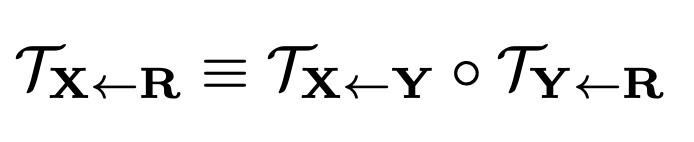Where `TX<-Y` is a transformation that deforms an image X to obtain an image Y. Thus, the keypoints of the original image X `Tx<-R` should be similar to the keypoints of the image Y `TY<-R`.

``````batch_size = driving_images.shape
transform = Transform(batch_size)

transformed_frame = transform.transform_frame(driving_images)

transformed_keypoints = self.key_point_detector(transformed_frame)

keypoints_loss = tf.reduce_mean(tf.abs(kp_driving['value'] - transform.warp_coordinates(transformed_keypoints['value'])))
``````

In the code above the method transform_frame from the class Transform applies the TPS to the driving image using the tfa.image.resampler() function.

We compute the keypoint locations for this new transformed image using our keypoint detector network. The warp_coordinates method applies the same TPS directly to the keypoints locations. The idea is that after two transformations, using transform_frame and then warp_coordinates, the keypoints should be similar or equivariant to the keypoints of the original image. We use the L1 loss function to compute the distance between the two keypoints.

We will also use this loss function to constrain the Jacobians. However, we need some changes.

First we have to extend the original equivariance constrain to include the Jacobians. This can be done using the Taylor expansion. We have to apply the first order Taylor expansion on both sides since the coefficients in both sides need to be equal.

The expansion of the left side is the same expansion as the motion equation expansion. In the right side we use the chain rule since we have two functions. You can see all the steps in the supplementary section of the paper. Then, the expansion looks like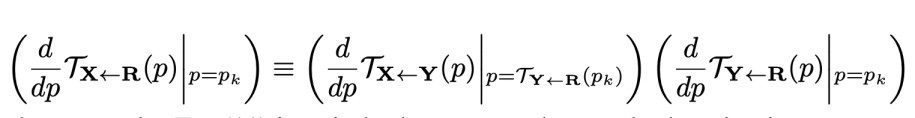That just means: the Jacobians of the original image should be similar to the Jacobians of the transformed image after the `TX<-Y` transformation.

However, using this equation leads to a problem where the use of L1 loss forces the magnitudes of the Jacobians to zero.

Thus, the authors reformulate the constraint in the following way: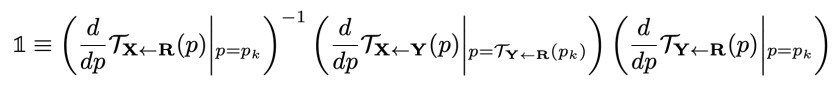Here, `1` is the identity matrix of shape `2x2`. We move the Jacobian `TX<-R` to the right side of the equation so now we have its inverse. The idea is that the Jacobian `TY<-R` after the transformation `TX<-Y` should have similar values than the original Jacobian `TX<-R`. Thus, `TX<-R` and `TY<-R` should have the same values. Computing the matrix multiplication of a matrix with its inverse returns the identity matrix:

``````M-1 . M = 1
TX<-R(-1)  . TY<-R = 1
``````

We ignored the d/dp notation.

``````jacobian_transformed = tf.linalg.matmul(transform.jacobian(transformed_keypoints['value']), transformed_keypoints['jacobian'])

normed_driving = tf.linalg.inv(kp_driving['jacobian'])
normed_transformed = jacobian_transformed

jacobian_mul = tf.linalg.matmul(normed_driving, normed_transformed)
identity_matrix = tf.cast(tf.reshape(tf.eye(2), [1, 1, 2, 2]), jacobian_mul.dtype)
jacobian_loss = tf.reduce_mean(tf.abs(identity_matrix - jacobian_mul))
``````

We can notice that we use the keypoints of the transformed image as the input of the method transform.jacobian. We can releate from the last equation that we have the transformation `TX<-Y`, and since we expanded this equation, we need its derivative evaluated at the keypoint location of the transformed image `TY<-R`.

The method transform.jacobian looks like:

``````def jacobian(self, coordinates):
new_coordinates = self.warp_coordinates(coordinates)
x = tf.keras.backend.sum(new_coordinates[..., 0])
y = tf.keras.backend.sum(new_coordinates[..., 1])

``````

Here we compute the transformation `TX<-Y` using the method warp_coordinates and compute its derivative with respect to x and y using the tape.gradient function.

In TensorFlow 2.0 when we want to train a model, in order to compute the gradients of the trainable variables like weights, we need to call all the models and functions inside a block of code like:

``````with tf.GradientTape() as tape:
``````

Thus, we indicate that we want to register all the operations inside this function so we can compute its gradients. We can use tape inside this block of code if we need to compute the gradients of some function. The computations of these gradients are also registered so it's a little bit expensive to call tape inside `tf.GradientTape()`.

In the case of PyTorch, we have a function called torch.autograd.grad that we can use to compute the gradients. PyTorch automatically registers the operations of the tensors which parameter requires_grad is set to True. Once we call the function backward, the framework uses these saved operations to compute the gradients and store them in tensor.grad. Using torch.autograd.grad we don't have to wait to call backward to obtain the gradients of some tensor.

Retaking the equivariance loss code. We use the L1 loss to compute the distance between the identity matrix and the multiplication between the inverse Jacobian and the transformed Jacobian. Thus, we don't apply the L1 loss directly to the Jacobians so we avoid the problems with the magnitudes.

## Full Discriminator

In the same way, we have a full discriminator model that consolidates several computations including the loss. In the original code, you may notice that the code for the discriminator is a little bit different as they use a discriminator that can accept multiple image scales. Here I simplified this model since that configuration is not always used.

``````class FullDiscriminator(tf.keras.Model):
def __init__(self):
super(FulDiscriminator, self).__init__()
self.discriminator = discriminator

def call(self, x_driving, generated):
kp_driving = generated['kp_driving']

loss_values = {}

_, discriminator_pred_map_real = self.discriminator(x_driving, kp=detach_keypoint(kp_driving))
_, discriminator_pred_map_generated = self.discriminator(tf.stop_gradient(generated['prediction']), kp=detach_keypoint(kp_driving))

discriminator_loss = (1 - discriminator_pred_map_real) ** 2 + discriminator_pred_map_generated ** 2

loss_values['disc_gan'] = tf.reduce_mean(discriminator_loss)

return loss_values
``````

Here we use the same discriminator that we use in the FullGenerator model. As we saw, we use a gaussian heatmap as input. This heatmap provides the keypoints locations to the discriminator so it can focus on the moving parts of the image.

We also stop the gradients of the keypoints and Jacobians so these are used as input images. The loss function is the well-known loss that we use to train the discriminator network where we want the outputs of the real images to be 1 or 0 for the generated images.

What I found interesting is the fact that the generator is able to learn the appearance of the source image only using the generator and discriminator losses as objective functions. The perceptual and equivariance losses are used to copy the content of the driving image and to localize good keypoints respectively, so these losses are not used to match the appearance or style of the image.

## Training

We use the FullGenerator and FullDiscriminator models to train all the networks in two steps. In the first step, we train all the networks inside the FullGenerator except the discriminator that we train in the second step using the FullDiscriminator model. Thus, we have to compute the gradients and apply the optimizers twice:

``````@tf.function
def train_step(source_images, driving_images):
with tf.GradientTape(persistent=True) as tape:
losses_generator, generated = generator_full(source_images, driving_images)
loss = tf.math.reduce_sum(list(losses_generator.values()))

with tf.GradientTape() as tape:
losses_discriminator = discriminator_full(x)
discriminator_loss = tf.math.reduce_sum(list(losses_discriminator.values()))

return loss
``````

We use Adam optimizer to train the generator, keypoint detector and discriminator. The dense estimator network is trained along with the generator. We use the following hyperparameters:

``````lr = 2e-4

optimizer_generator = tf.keras.optimizers.Adam(learning_rate=lr, beta_1=0.5, beta_2=0.999)
optimizer_keypoint_detector = tf.keras.optimizers.Adam(learning_rate=lr, beta_1=0.5, beta_2=0.999)
optimizer_discriminator = tf.keras.optimizers.Adam(learning_rate=lr, beta_1=0.5, beta_2=0.999)

batch_size = 20
epochs = 150
``````

We can use several datasets to train these models. All depedents of what we want to achieve. We can find the instructions to get and preprocess these datasets here. Of course, to preprocess and train these models we need a lot of power or in other words, some good GPU's.

## Conclusion

In this post we have seen how we can anime an object in an image using the motion of frames from some video. In the process we learn about Optical Flow, Taylor Expansion, Bilinear Interpolation, Self-supervised keypoints and some other methods. This post still can be expanded and improved. For example, we could get a better explanation of how the thin plate spline transformations work and how they are built.